# Frobenius algebra

(diff) ← Older revision | Latest revision (diff) | Newer revision → (diff)

A finite-dimensional algebraover a fieldsuch that the left-modulesand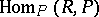are isomorphic. In the language of representations this means that the left and right regular representations are equivalent. Every group algebra of a finite group over a field is a Frobenius algebra. Every Frobenius algebra is a quasi-Frobenius ring. The converse is not true. The following properties of a finite-dimensional-algebraare equivalent:

1)is a Frobenius algebra;

2) there is a non-degenerate bilinear form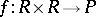such that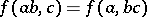for all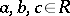;

3) ifis a left andis a right ideal of, then (see Annihilator)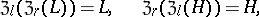Frobenius algebras essentially first appeared in the papers of G. Frobenius .

How to Cite This Entry:
Frobenius algebra. Encyclopedia of Mathematics. URL: http://encyclopediaofmath.org/index.php?title=Frobenius_algebra&oldid=14845
This article was adapted from an original article by L.A. Skornyakov (originator), which appeared in Encyclopedia of Mathematics - ISBN 1402006098. See original article